Updating search results...

# 20 Results

View
Selected filters:
• circle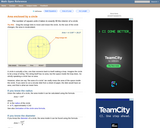Rating
0.0 stars

An interactive applet and associated web page that demonstrate the area of a circle. A circle is shown with a point on the circumference that can be dragged to resize the circle. As the circle is resized, the radius and the area computation is shown changing in real time. The radius and formula can be hidden for class discussion. Applet can be enlarged to full screen size for use with a classroom projector. This resource is a component of the Math Open Reference Interactive Geometry textbook project at http://www.mathopenref.com.

Subject:
Geometry
Mathematics
Material Type:
Simulation
Provider:
Math Open Reference
Author:
John Page
05/07/2019Rating
0.0 stars

Students compare the relationships between radius, diameter, circumference, and area by manipulating a circle and increasing or decreasing its size in this interactive from Illuminations. It also provides a problems section where students determine the answer to each question using the applet, a calculator, or paper and pencil. They must also indicate the proper units!

Subject:
Geometry
Mathematics
Material Type:
Activity/Lab
Interactive
Provider:
National Council of Teachers of Mathematics
Author:
Illuminations
02/26/2019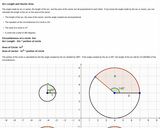Unrestricted Use
CC BY
Rating
0.0 stars

Proportionality between circumference, angle and arc length; area, angle and area of sector. Circumference and area of circle equation, Image of four circles with hotpots of calculations

Subject:
Geometry
Mathematics
Material Type:
Interactive
Provider:
Michigan Virtual
07/19/2019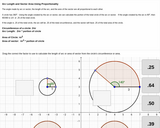Unrestricted Use
CC BY
Rating
0.0 stars

Angle of sector or arc is proportional to the circumference or area of total circle. Interactive drag and drop factor to use when calculating arc length or sector area.

Subject:
Geometry
Mathematics
Material Type:
Interactive
Provider:
Michigan Virtual
07/19/2019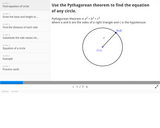Unrestricted Use
CC BY
Rating
0.0 stars

Slides showing steps to derive the equation of a circle, using the Pythagorean Theorem

Subject:
Geometry
Mathematics
Material Type:
Interactive
Provider:
Michigan Virtual
07/19/2019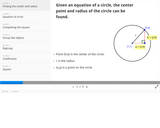Unrestricted Use
CC BY
Rating
0.0 stars

Slides showing steps to solve for center and radius of a circle given an equation of a circle by completing the square.

Subject:
Geometry
Mathematics
Material Type:
Interactive
Provider:
Michigan Virtual
07/19/2019Rating
0.0 stars

The Greek mathematician Archimedes approximated pi by inscribing and circumscribing polygons about a circle and calculating their perimeters. Similarly, the value of pi can be approximated by calculating the areas of inscribed and circumscribed polygons. This activity allows for the investigation and comparison of both methods.

Subject:
Mathematics
Material Type:
Activity/Lab
Interactive
Provider:
National Council of Teachers of Mathematics
Author:
Illuminations
02/26/2019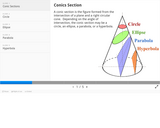Unrestricted Use
CC BY
Rating
0.0 stars

Image showing four conic sections, with an example of each type of equation; circle, ellipse, parabola, and hyperbola.

Subject:
Geometry
Mathematics
Material Type:
Interactive
Provider:
Michigan Virtual
07/19/2019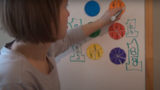Unrestricted Use
CC BY
Rating
0.0 stars

This lesson is Day 2 in a series of 12 lessons around fraction equivalences and comparisons.

This video lesson introduces the idea that fractions can be used to represent 1 whole. To engage in this lesson, students may use virtual circle models from Toy Theater (https://toytheater.com/fraction-circles/)

Subject:
Mathematics
Material Type:
Activity/Lab
Interactive
Lesson
Simulation
Author:
Dawne Coker
06/25/2020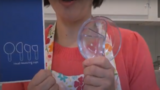Unrestricted Use
CC BY
Rating
0.0 stars

This lesson is Day 4 in a series of 12 lessons around fraction equivalences and comparisons.

This video lesson looks at how we use fractions in the kitchen. Students are challenged to use circle models to find equivalent fractions for a recipe. Students may use virtual circe models from the Toy Theater (https://toytheater.com/fraction-circles/).

Subject:
Mathematics
Material Type:
Activity/Lab
Interactive
Lesson
Simulation
Author:
Dawne Coker
06/25/2020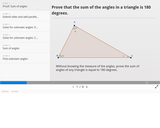Unrestricted Use
CC BY
Rating
0.0 stars

Definition of circle and similarity, images of circles shown to map to one another after a translation and dilation. Interactive word drag of keywords of steps of proof.

Subject:
Geometry
Mathematics
Material Type:
Interactive
Provider:
Michigan Virtual
07/19/2019Rating
0.0 stars

Lesson plan where students are asked to consider how to draw a perfect circle, and learn to do so using a line segment called a radius. They also learn to fold a circle in two directions symmetrically to find its center. This activity is motivated by a CYBERCHASE episode in which the CyberSquad set out to rescue a magic ring from Hacker and deposit it in a safe place.

Subject:
Geometry
Mathematics
Material Type:
Activity/Lab
Lesson Plan
Presentation
Provider:
WGBH - Teachers' Domain
Author:
WNET.org
02/26/2019Rating
0.0 stars

In this video segment from Cyberchase, the CyberSquad is trapped in one of the Spheres of Fears, and they are trying to get through a gate to escape. The key to the gate is locked inside a can, and in order to access the key they must attach the correct label to the can. After guessing incorrectly on the first try, the CyberSquad decides to take some measurements to find the correct label. Using two circles nearby, they compare the diameter of each circle to its circumference. They realize there is a special relationship between the circumference of a circle and the length of its diameter. Teaching tips are also provided that discuss frame, focus and follow-up suggestions for using this video in a math lesson.

Subject:
Geometry
Mathematics
Material Type:
Activity/Lab
Presentation
Provider:
WGBH - Teachers' Domain
Author:
WNET.org
02/26/2019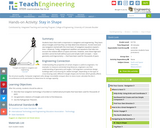Educational Use
Rating
0.0 stars

Students learn that math is important in navigation and engineering. They learn about triangles and how they can help determine distances. Ancient land and sea navigators started with the most basic of navigation equations (speed x time = distance). Today, navigational satellites use equations that take into account the relative effects of space and time. However, even these high-tech wonders cannot be built without pure and simple math concepts â basic geometry and trigonometry â that have been used for thousands of years.

Subject:
Applied Science
Engineering
Material Type:
Activity/Lab
Provider:
TeachEngineering
Provider Set:
TeachEngineering
Author:
Janet Yowell
Jeff White
Malinda Schaefer Zarske
Matt Lippis
10/14/2015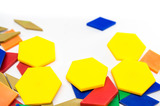Unrestricted Use
CC BY
Rating
0.0 stars

This resource is from Tools4NCTeachers.In this lesson, students explore attributes of polygons in order to create 2D shapes using given attributes.&nbsp; Students need some basic background knowledge of the attributes of polygons, including angles, sides, vertices, and right angles.&nbsp;&nbsp;Remix this lesson to include sample student work... Sharing is caring :)

Subject:
Mathematics
Material Type:
Activity/Lab
Lesson
Lesson Plan
Author:
DAWNE COKER
06/24/2020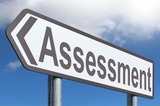Unrestricted Use
CC BY
Rating
0.0 stars

This resource is from Tools4NCTeachers.A Common Formative Assessment (CFA) provides a snapshot of current student understanding. It serves as a checkpoint, and may be used to guide future whole and small group instruction. This CFA addresses 2nd grade, Cluster 9&nbsp;standards. &nbsp;Answer key is provided.

Subject:
Mathematics
Material Type:
Assessment
Formative Assessment
Author:
DAWNE COKER
06/16/2020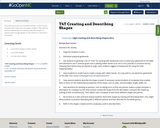Conditional Remix & Share Permitted
CC BY-NC-SA
Rating
0.0 stars

This resource is part of Tools4NCTeachers.

In this lesson, students will create and name given shapes. They will use precise mathematical vocabulary describe shapes to partner with enough details for the partner to recreate it accurately.

Subject:
Mathematics
Material Type:
Lesson
Lesson Plan
11/06/2019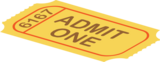Unrestricted Use
CC BY
Rating
0.0 stars

This resource is part of Tools4NCTeachers.This packet contains exit tickets for the First Grade stnanard G.3 (partitioning shapes). Exit tickets are written responses to questions posed at the end of a lesson. They are brief assessments which allow the teacher to determine student understanding of the concepts and skills taught that day.&nbsp;

Subject:
Mathematics
Material Type:
Assessment
Formative Assessment
Self Assessment
Author:
DAWNE COKER
06/14/2020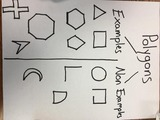Unrestricted Use
CC BY
Rating
0.0 stars

This resource is from Tools4NCTeachers. This file contains sample anchor charts to create with students. Anchor charts are to be made with students, then displayed as a classroom reference. Parents may also benefit from seeing anchor charts, as they provide a snapshot into the math class.Remix this resource to include pictures of your own anchor charts.

Subject:
Mathematics
Material Type:
Activity/Lab
Diagram/Illustration
Reference Material
Author:
DAWNE COKER
06/21/2020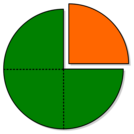Unrestricted Use
CC BY
Rating
0.0 stars

This resource is from Tools4NCTeachers.&nbsp;&nbsp;In this lesson students are introduced to the vocabulary associated with partitioning shapes into equal shares. Students will also explore shapes that are partitioned into halves, thirds, and fourths. Student recording sheets and pictures of anchor charts are included within this lesson.&nbsp;

Subject:
Mathematics
Material Type:
Activity/Lab
Lesson
Lesson Plan
Author:
DAWNE COKER